# How Exponential Smoothing Forecast works

The Exponential Smoothing Forecast tool uses the Holt-Winters exponential smoothing method to decompose the time series at each location of a space-time cube into seasonal and trend components to effectively forecast future time steps at each location. The primary output is a map of the final forecasted time step as well as informative messages and pop-up charts. You can also create a new space-time cube containing the data from the original cube along with the forecasted values appended. Additionally, you have the option to detect outliers in each time series to identify locations and times that significantly deviate from the patterns and trends of the rest of the time series.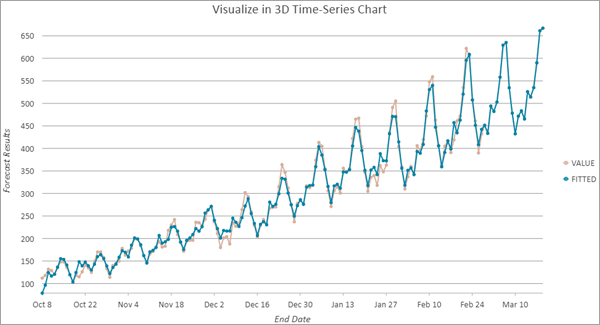## Potential applications

Exponential smoothing is one of the oldest and most studied time series forecasting methods. It is most effective when the values of the time series follow a gradual trend and display seasonal behavior in which the values follow a repeated cyclical pattern over a given number of time steps.

For example, you can use this tool in the following applications:

• A city health planner can use this tool to predict the hourly temperature of a city center during a heat wave to prepare for heat-related illnesses.
• A retail chain can use this tool to predict demand for individual items for each day of the following week.

## Forecasting and validation

The tool builds two models while forecasting each time series. The first is the forecast model, which is used to forecast the values of future time steps. The second is the validation model, which is used to validate the forecasted values.

### Forecast model

The forecast model is constructed by performing exponential smoothing on the time series values at each location of the space-time cube. This model is then used to forecast the future time steps. The fit of the exponential smoothing model to each time series is measured by the Forecast root mean square error (RMSE), which is equal to the square root of the average squared difference between the exponential smoothing model and the values of the time series.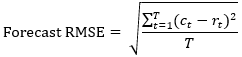, where T is the number of time steps, ct is the fitted value from exponential smoothing, and rt is the raw value of the time series at time t.

The following image shows the raw values of a time series and an exponential smoothing model fitted to the time series along with forecasts for two future time steps. The Forecast RMSE measures how much the fitted values from the model differ from the raw time series values.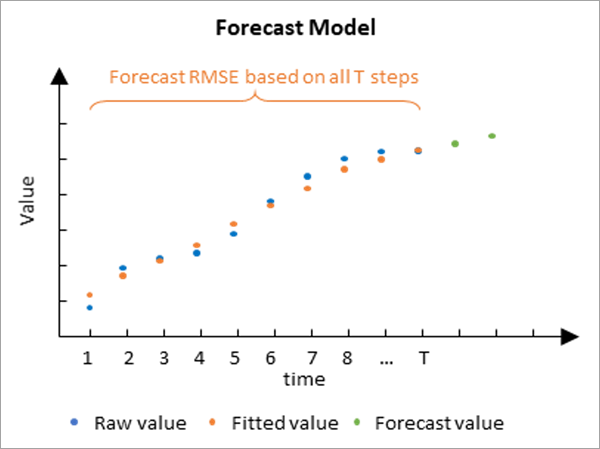The Forecast RMSE only measures how well the exponential smoothing model fits the raw time series values. It does not measure how well the forecast model actually forecasts future values. It is common for models to fit a time series closely but not provide accurate forecasts when extrapolated. This problem is addressed by the validation model.

### Validation model

The validation model is used to determine how well the forecast model can forecast future values of each time series. It is constructed by excluding some of the final time steps of each time series and fitting the exponential smoothing model to the data that was not excluded. This model is then used to forecast the values of the data that were withheld, and the forecasted values are compared to the raw values that were hidden. By default, 10 percent of the time steps are withheld for validation, but this number can be changed using the Numer of Time Steps to Exclude for Validation parameter. The number of time steps excluded cannot exceed 25 percent of the number of time steps, and no validation is performed if 0 is specified. The accuracy of the forecasts is measured by calculating a Validation RMSE statistic, which is equal to the square root of the average squared difference between the forecasted and raw values of the excluded time steps.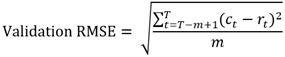, where T is the number of time steps, m is the number of time steps withheld for validation, ct is the value forecasted from the first T-m time steps, and rt is the raw value of the time series withheld for validation at time t.

The following image shows an exponential smoothing model fitted to the first half of a time series and used to predict the second half of the time series. The Validation RMSE measures how much the forecasted values differ from the raw values at the withheld time steps.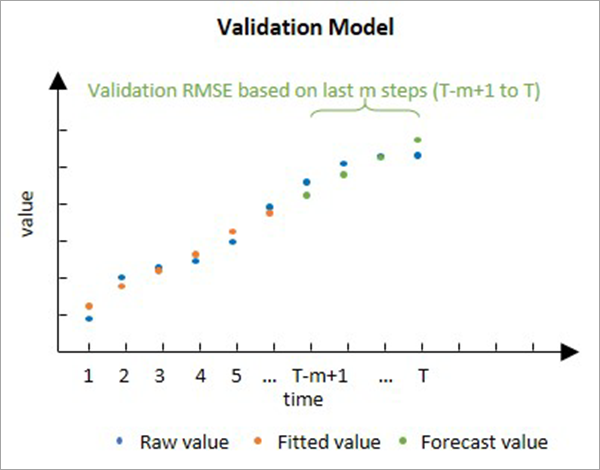The validation model is important because it can directly compare forecasted values to raw values to measure how well the exponential smoothing model can forecast. While it is not actually used to forecast, it is used to justify the forecast model.

##### Note:

Validation in time series forecasting is similar but not identical to a common technique called cross validation. The difference is that forecasting validation always excludes the final time steps for validation, and cross validation either excludes a random subset of the data or excludes each value sequentially.

### Interpretation

There are several considerations when interpreting the Forecast RMSE and Validation RMSE values.

• The RMSE values are not directly comparable to each other because they measure different things. The Forecast RMSE measures the fit of the model to the raw time series values, and the Validation RMSE measures how well the model can forecast future values. Because the Forecast RMSE uses more data and does not extrapolate, it is usually smaller than the Validation RMSE.
• Both RMSE values are in the units of the data. For example, if your data is temperature measurements in degrees Celsius, a Validation RMSE of 50 is very high because it means that the forecasted values differed from the true values by approximately 50 degrees on average. However, if your data is daily revenue in U.S. dollars of a large retail store, the same Validation RMSE of 50 is very small because it means that the forecasted daily revenue only differed from the true values by \$50 per day on average.

## Building the exponential smoothing model

There are various kinds of exponential smoothing, but they all work by separating the time series into several components. The values of each component are estimated by exponentially weighting the components from previous time steps such that the influence of each time step decreases exponentially going forward in time. Each component is defined recursively through a state-space model approach, and each component depends on all of the other components. All parameters are estimated using maximum likelihood estimation.

In this tool, all components are additive such that the forecast model is the sum of the individual components. If a seasonal component is used, the tool uses the Holt-Winters Damped Seasonal method. If no seasonal component is used, the tool uses the Damped Trend method. You can find the details of these components and the equations defining the state-space models in the textbook in the Additional references section.

### Trend component

The first component of the exponential smoothing model is the trend component. This component is used to model gradual and systematic changes in the values of the time series. It is estimated by exponentially weighting the values of each time step by the difference between its value and the value of the previous time step. The trend component is used directly when making forecasts using the last trend detected by the model. However, to prevent the forecasts from following the final trend forever, the trend is damped so that the trend gradually flattens going forward in time. Damping flattens the trend by multiplying the slope of the trend value at each time step by an exponentially decreasing value. The level of damping is estimated by the model, so the trend may flatten more quickly or slowly (or not flatten at all in the most extreme case) for some models than others when forecasting farther into the future.

### Seasonal component

The second component of exponential smoothing is the seasonal component, which is used to model patterns in the data that repeat over a given number of time steps. The shape and magnitude of the pattern within each season can change over time, but the duration of one season must be the same for the entire time series. For example, temperature displays seasonal behavior corresponding to days and nights with lowest temperatures during the night and highest temperatures during the day. While the sun may rise at different times of the day throughout the year (and thus change the shape and magnitude of the temperature pattern within a single day), the duration of a season is always one day.

As with the trend component, the seasonal component of a given time step is determined by exponentially weighting the seasonal values of the previous time steps. However, instead of using the time steps immediately before, it only weights the previous time steps corresponding to the same point in a seasonal cycle. For example, if the length of a season is four time steps, the seasonal component exponentially weights the values 4 time steps prior, 8 time steps prior, 12 time steps prior, and so on.

If you know the number of time steps that correspond to one season in your data, you can specify it in the Season Length parameter, and this value will be used by every location in the space-time cube. If you do not know the length of a season or if the seasonal length is different for different locations, the parameter value can be left empty, and an optimal season length will be estimated for each location using a spectral density function. For details about this function, see the Additional resources section.

For an individual location, if the optimal season length determined by spectral analysis is greater than one and less than one-third of the number of time steps at the location, the season length is set to this optimal value. Otherwise, the location does not use a seasonal component. The season length used at the location is saved in the Season Length field of the output features. If a seasonal component is not used, the value in this field is 1. This workflow is summarized in the following image: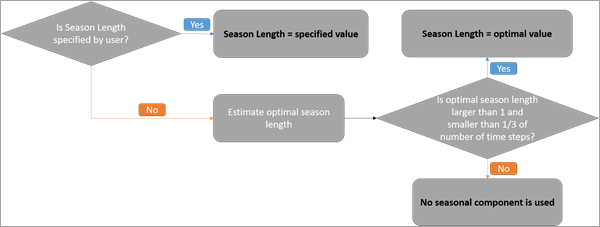### Level component

The level component of exponential smoothing represents the baseline value of the time series taking into account the seasonality and trend. When fitting the forecast model to the input space time cube, the level of a time step is computed by exponentially weighting the previous levels while factoring in the seasonality and trend. When forecasting to the future, the level component stays equal to the level component of the final measured time step, and the actual forecasts are instead driven by the trend and seasonal components.

### Residual component and confidence intervals

The final component is the residual (or error) component. This component is the difference between the true value and the value estimated by all other components. It represents the remaining uncertainty and error in the data after the trend, season, and level components have been modeled. This component is important because it forms the basis for confidence intervals.

For each forecasted time step, the tool computes upper and lower bounds of a 90 percent confidence interval for the forecasted value. The forecasted value at each time step represents the single best estimate for the future value, but the confidence interval can be used to visualize the uncertainty and likely range of the true future value. The upper and lower bounds are saved as fields and displayed in pop-up charts of the Output Features.

The confidence intervals are estimated by assuming that the residuals of the model are independently and identically normally distributed. Under this assumption, formulas for the confidence intervals can be derived. These formulas and their derivations can be found in the textbook in the Additional references section.

### Visualize the components

You can visualize the components of your exponential smoothing model by creating an output space-time cube. Use this cube in the Visualize Space Time Cube in 3D tool with the Forecast results option for the Display Theme parameter. A chart is created for the output features, and the various components of the exponential smoothing model can be turned on and off in the Chart Properties pane. When these components are added together, they construct the forecast model and the forecasts for the future time steps. The following image shows the individual components of the exponential smoothing model shown in the first image of this topic: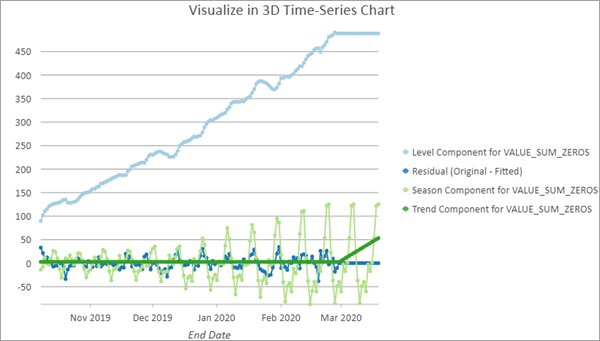## Identifying time series outliers

Outliers in time series data are values that significantly differ from the patterns and trends of the other values in the time series. For example, large numbers of online purchases around holidays or high numbers of traffic accidents during heavy rainstorms would likely be detected as outliers in their time series. Simple data entry errors, such as omitting the decimal of a number, are another common source of outliers. Identifying outliers in time series forecasting is important because outliers influence the forecast model that is used to forecast future values, and even a small number of outliers in the time series of a location can significantly reduce the accuracy and reliability of the forecasts. Locations with outliers, particularly outliers toward the beginning or end of the time series, may produce misleading forecasts, and identifying these locations helps you determine how confident you should be in the forecasted values at each location.

Outliers are not determined simply by their raw values but instead by how much their values differ from the fitted values of the forecast model. This means that whether or not a value is determined to be an outlier is contextual and depends both on its place and time. The forecast model defines what the value is expected to be based on the entire time series, and outliers are the values that deviate significantly from this baseline. For example, consider a time series of annual mean temperature. Because average temperatures have increased over the last several decades, the fitted forecast model of temperature will also increase over time to reflect this increase. This means that a temperature value that would be considered typical and not an outlier in 1950 would likely be considered an outlier if the same temperature occurred in 2020. In other words, a typical temperature from 1950 would be considered very low by the standards of 2020.

You can choose to detect time series outliers at each location using the Identify Outliers parameter. If specified, the Generalized Extreme Studentized Deviate (ESD) test is performed for each location to test for time series outliers. The confidence level of the test can be specified with the Level of Confidence parameter, and 90 percent confidence is used by default. The Generalized ESD test iteratively tests for a single outlier, two outliers, three outliers, and so on, at each location up to the value of the Maximum Number of Outliers parameter (by default, 5 percent of the number of time steps, rounded down), and the largest statistically significant number of outliers is returned. The number of outliers at each location can be seen in the attribute table of the output features, and individual outliers can be seen in the time series pop-up charts that are discussed in the next section.

Learn more about outliers in time series analysis, the Generalized ESD test, and how to interpret the results

## Tool outputs

The primary output of this tool is a 2D feature class showing each location in the Input Space Time Cube symbolized by the final forecasted time step with the forecasts for all other time steps stored as fields. Although each location is independently forecasted and spatial relationships are not taken into account, the map may display spatial patterns for areas with similar time series.

### Pop-up charts

Clicking any feature on the map using the Explore navigation tool displays a chart in the Pop-up pane showing the values of the space-time cube along with the fitted exponential smoothing model and the forecasted values along with 90 percent confidence intervals for each forecast. The values of the space-time cube are displayed in blue and are connected by a blue line. The fitted values are displayed in orange and are connected by a dashed orange line. The forecasted values are displayed in orange and are connected by a solid orange line representing the forecasting of the model. Light red confidence bounds are drawn around each forecasted value. You can hover over any point in the chart to see the date and value of the point. Additionally, if you chose to detect outliers in time series, any outliers are displayed as large purple dots.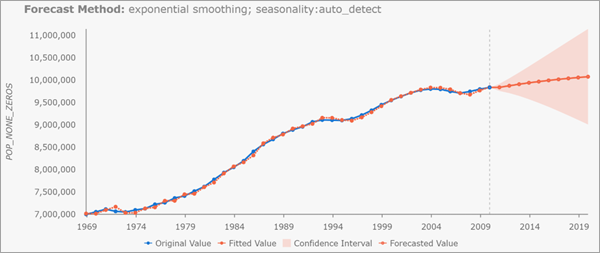##### Note:

Pop-up charts are not created when the output features are saved as a shapefile (.shp).

### Geoprocessing messages

The tool provides a number of messages with information about the tool execution. The messages have three main sections.

The Input Space Time Cube Details section displays properties of the input space-time cube along with information about the number of time steps, number of locations, and number of space-time bins. The properties displayed in this first section depend on how the cube was originally created, so the information varies from cube to cube.

The Analysis Details section displays properties of the forecast results, including the number of forecasted time steps, the number of time steps excluded for validation, the percent of locations with seasonality, and information about the forecasted time steps. If a value is not provided for the Season Length parameter, summary statistics of the estimated season lengths are displayed, including the minimum, maximum, average, median, and standard deviation.

The Summary of Accuracy across Locations section displays summary statistics for the Forecast RMSE and Validation RMSE among all of the locations. For each value, the minimum, maximum, mean, median, and standard deviation is displayed.

The Summary of Time Series Outliers section appears if you choose to detect time series outliers using the Outlier Option parameter. This section displays information including the number and percent of locations containing outliers, the time step containing the most outliers, and summary statistics for the number of outliers by location and by time step.

##### Note:

Geoprocessing messages appear at the bottom of the Geoprocessing pane during tool execution. You can access the messages by hovering over the progress bar, clicking the pop-out button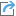, or expanding the messages section in the Geoprocessing pane. You can also access the messages for a previously run tool using geoprocessing history.

### Fields of the output features

In addition to Object ID, geometry fields, and the field containing the pop-up charts, the Output Features will have the following fields:

• Location ID (LOCATION)—The Location ID of the corresponding location of the space-time cube.
• Forecast for (Analysis Variable) in (Time Step) (FCAST_1, FCAST_2, and so on)—The forecasted value of each future time step. The field alias displays the name of the Analysis Variable and the date of the forecast. A field of this type is created for each forecasted time step.
• High Interval for (Analysis Variable) in (Time Step) (HIGH_1, HIGH_2, and so on)—The upper bound of a 90 percent confidence interval for the forecasted value of each future time step. The field alias displays the name of the Analysis Variable and the date of the forecast. A field of this type is created for each forecasted time step.
• Low Interval for (Analysis Variable) in (Time Step) (LOW_1, LOW_2, and so on)—The lower bound of a 90 percent confidence interval for the forecasted value of each future time step. The field alias displays the name of the Analysis Variable and the date of the forecast. A field of this type is created for each forecasted time step.
• Forecast Root Mean Square Error (F_RMSE)—The Forecast RMSE.
• Validation Root Mean Square Error (V_RMSE)—The Validation RMSE. If no time steps were excluded for validation, this field is not created.
• Season Length (SEASON)—The number of time steps corresponding to one season for the location. A value of 1 in this field means there is no seasonality.
• Forecast Method (METHOD)—A text field displaying the model used at the location. For this tool, the value is always exponential smoothing. This field allows you to identify which models are used in the Evaluate Forecasts By Location tool.
• Number of Model Fit Outliers (N_OUTLIERS)—The number of outliers detected in the time series of the location. This field is only created if you chose to detect outliers with the Outlier Option parameter.

### Output space-time cube

If an Output Space Time Cube is specified, the output cube contains all of the original values from the input space-time cube with the forecasted values appended. This new space-time cube can be displayed using the Visualize Space Time Cube in 2D or Visualize Space time Cube in 3D tools and can be used as input to the tools in the Space Time Pattern Mining toolbox, such as Emerging Hot Spot Analysis and Time Series Clustering.

Multiple forecasted space-time cubes can be compared and merged using the Evaluate Forecasts by Location tool. This allows you to create multiple forecast cubes using different forecasting tools and parameters, and the tool identifies the best forecast for each location using either Forecast or Validation RMSE.

## Best practices and limitations

When deciding whether this tool is appropriate for your data and which parameters you should choose, several things should be taken into account.

• Compared to other forecasting tools in the Time Series Forecasting toolset, this tool is recommended for data that has moderate trends and strong seasonal behavior. The exponential model assumes that the seasonal behavior and the trend can be separated, so it is most effective for data whose trend changes gradually and follows consistent seasonal patterns over time. The seasonal component of the model is optional, so this tool can be used for data that does not display seasonality, but it performs best for strong seasonal behavior.
• Deciding how many time steps to exclude for validation is important. The more time steps are excluded, the fewer time steps remain to estimate the validation model. However, if too few time steps are excluded, the Validation RMSE is estimated using a small amount of data and may be misleading. It is recommended that you exclude as many time steps as possible while still maintaining sufficient time steps to estimate the validation model. It is also recommended that you withhold at least as many time steps for validation as the number of time steps you intend to forecast, if your space-time cube has enough time steps to allow this.